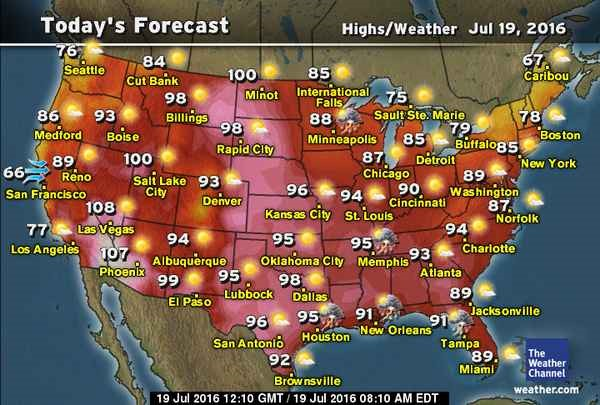##### KAPEC GEOTASK?
• The learning process is entertaining and exciting
• The solved tasks turn into coordinates
• The chance to follow your progress
• Along with your schoolmates and friends, go on an adventure, searching for hideouts
• E-mail:

# 109.1. Heat and temperature.

#### Where on the map temperature is equal with 303,15 K?#### Next task: 1324. Heat and temperature.

Grade:
8
Subject
Physics
Theme
Heat process
Subtheme
Heat and temperature.

#### Solve tasks to find hidden Geo point coordinates

1. Physics: The spread of sound.
2. Physics: Interaction, strength and safety.
3. Chemistry: Gas volume calculations.
4. Geometry: Triangles and trapezes midline.
5. Geometry: Rectangular (S = ab, a, b - edges) and area of the square (S=a^2 , a - edge) formula.
6. Algebra: Quadratic equation discriminant
7. Chemistry: Chemical element oxidation degree. Binary compound formula and name.
8. Algebra: Transformation of algebraic expressions.
9. Algebra: Actual numbers.
10. Chemistry: Acids, bases and salts chemical formulas and names.